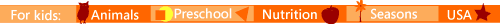Math Man Math Games
Mixed OperationsAddictive and fun arcade math games!
avoid ghosts and sharpen your
math skills all at the same time.
Mathman Mathman ExpertUp to 10
Up to 20 Up to 50 Up to 100

Subtractto 10 to 20 to 50 to 100

Multiply
Divisionto 10 to 12 to 64 Divide to 50

FractionsEquivalent Fractions
Mathman
Reduce Fractions
Mathman
Convert Improper
to Mixed Numbers
Same Denominator
Unlike Denominator
DecimalsFractions of a Round Mixed Fractions on a Decimal Number Line
Number Numbers Number Line

Place Value Time MoneyElapsed Time

IntegersUnderstand Integers Integers
On Number Line
Estimate Integers
On Number Line

Round & EstimateRound Hundreds Round Thousands Round Millions Estimate Numbers
On Number Line
EstimateSums
By RoundingEstimate Products
By Rounding
Round Mixed
Numbers
Round Money
Amounts
Estimate Integers
On Number Line